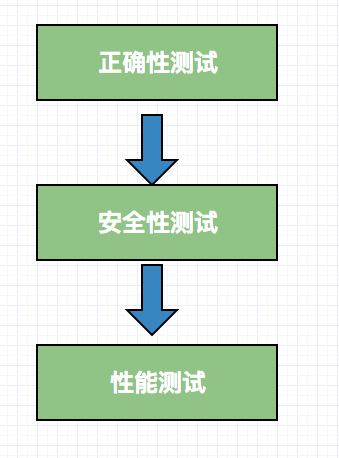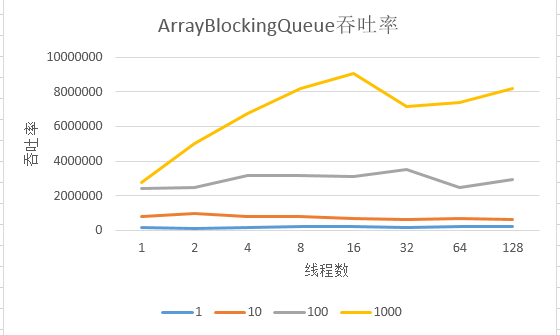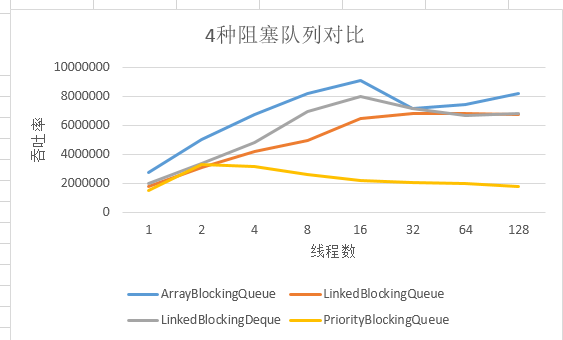java多线程——并发测试

cool_wier——————————————————————–

1. 并发测试分类2. 正确性测试

3. 安全性测试

4. 性能测试

12

public class PutTakeTest {

protected static final ExecutorService pool = Executors.newCachedThreadPool();

//栅栏，通过它可以实现让一组线程等待至某个状态之后再全部同时执行

protected CyclicBarrier barrier;

protected final ArrayBlockingQueue<Integer> bb;

protected final int nTrials, nPairs;

//入列总和

protected final AtomicInteger putSum = new AtomicInteger(0);

//出列总和

protected final AtomicInteger takeSum = new AtomicInteger(0);

public static void main(String[] args) throws Exception {

new PutTakeTest(10, 10, 100000).test(); // 10个承载因子，10个线程，运行100000

pool.shutdown();

}

public PutTakeTest(int capacity, int npairs, int ntrials) {

this.bb = new ArrayBlockingQueue<Integer>(capacity);

this.nTrials = ntrials;

this.nPairs = npairs;

this.barrier = new CyclicBarrier(npairs * 2 + 1);

}

void test() {

try {

for (int i = 0; i < nPairs; i++) {

pool.execute(new Producer());

pool.execute(new Consumer());

}

barrier.await(); // 等待所有的线程就绪

barrier.await(); // 等待所有的线程执行完成

System.out.println(“result，put==take :”+(putSum.get()==takeSum.get()));

} catch (Exception e) {

throw new RuntimeException(e);

}

}

static int xorShift(int y) {

y ^= (y << 6);

y ^= (y >>> 21);

y ^= (y << 7);

return y;

}

//生产者

class Producer implements Runnable {

public void run() {

try {

int seed = (this.hashCode() ^ (int) System.nanoTime());

int sum = 0;

barrier.await();

for (int i = nTrials; i > 0; –i) {

bb.put(seed);

sum += seed;

seed = xorShift(seed);

}

barrier.await();

} catch (Exception e) {

throw new RuntimeException(e);

}

}

}

//消费者

class Consumer implements Runnable {

public void run() {

try {

barrier.await();

int sum = 0;

for (int i = nTrials; i > 0; –i) {

sum += bb.take();

}

barrier.await();

} catch (Exception e) {

throw new RuntimeException(e);

}

}

}

}

for (int i = nTrials; i > 0; –i) {

bb.put(seed);

sum += seed;

seed = xorShift(seed);

}

3

1、为了保证时间精确性，增加一个运行一组操作的运行时间。

public class BarrierTimer implements Runnable{

private boolean started;

private long startTime, endTime;

public synchronized void run() {

long t = System.nanoTime();

if (!started) {

started = true;

startTime = t;

} else

endTime = t;

}

public synchronized void clear() {

started = false;

}

public synchronized long getTime() {

return endTime – startTime;

}

}

2、性能测试需要针对不同参数组合进行测试。

public static void main(String[] args) throws Exception {

int tpt = 100000; // trials per thread

for (int cap = 1; cap <= 1000; cap *= 10) {

System.out.println(“Capacity: ” + cap);

for (int pairs = 1; pairs <= 128; pairs *= 2) {

TimedPutTakeTest t = new TimedPutTakeTest(cap, pairs, tpt);

System.out.print(“Pairs: ” + pairs + “\t”);

t.test();

System.out.print(“\t”);

t.test();

System.out.println();

}

}

PutTakeTest.pool.shutdown();

}

CPU： i7 4核8线程memory：  16G

jdk 环境

java version “1.8.0_45″

Java HotSpot(TM) 64-Bit Server VM (build 25.45-b02, mixed mode)1、在ArrayBlockingQueue的缓存容量在1的情况下，无论线性并发数为多少，都不能显著的提升其吞吐率。这是因为每个线程在阻塞等待另外线程执行任务。2、当尝试把缓存容量提升至10、100、1000的时候，吞吐率都得到了极大的提高，特别是在1000的时候，最高可达到900w次/s。

3、当线程增加到16个的时候，吞吐率会达到顶峰，然后再增加线程吞吐率不生反而下降，当然没有很大的下降，这是因为，当线程增多的时候，大部分时间耗费在阻塞和解除阻塞上面了。4

end